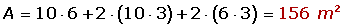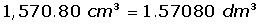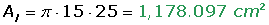Learn from home

The teachers## Exercise 1

Calculate the volume (in cubic centimeters) of a prism that is 5 m long, 40 cm wide and 2500 mm high.

## Exercise 2

A swimming pool is 8 m long, 6 m wide and 1.5 m deep. The water resistant paint needed for the pool costs300 per m², what is the total cost of the restoration?

## Exercise 10

How many square tiles (20 cm x 20 cm) are needed to coat the sides and base of a pool which is 10 m long, 6 meters wide and 3 m deep?

## Exercise 11

A cylindrical container with a radius of 10 cm and a height of 5 cm is filled with water. If the total mass of the filled container is 2 kg, what is the mass of the empty container?

## Exercise 12

For a party, Louis has made about 10 conical hats out of cardboard. How much cardboard was used in total if each cap has a radius of 15 cm and a slant height of 25 cm?

## Exercise 13

A cube with an edge of 20 cm is filled with water. Would this amount of water fit in a sphere with a 20 cm radius?

## Solution of exercise 1

Calculate the volume (in cubic centimeters) of a prism that is 5 m long, 40 cm wide and 2,500 mm high.## Solution of exercise 2

A swimming pool is 8 m long, 6 m wide and 1.5 m deep. The water resistant paint needed for the pool costs300 per m², what is the total cost of the restoration?15,707.96 · 300 = \$4,712,388.98

## Solution of exercise 10

How many square tiles (20 cm x 20 cm) are needed to coat the sides and base of a pool which is 10 m long, 6 meters wide and 3 m deep?## Solution of exercise 11

A cylindrical container with a radius of 10 cm and a height of 5 cm is filled with water. If the total mass of the filled container is 2 kg, what is the mass of the empty container?## Solution of exercise 12

For a party, Louis has made about 10 conical hats out of cardboard. How much cardboard was used in total if each cap has a radius of 15 cm and a slant height of 25 cm?## Solution of exercise 13

A cube with an edge of 20 cm is filled with water. Would this amount of water fit in a sphere with a 20 cm radius?Did you like the article?(1 votes, average: 5.00 out of 5)Loading...

Emma

I am passionate about travelling and currently live and work in Paris. I like to spend my time reading, gardening, running, learning languages and exploring new places.

Did you like
this resource?

Bravo!